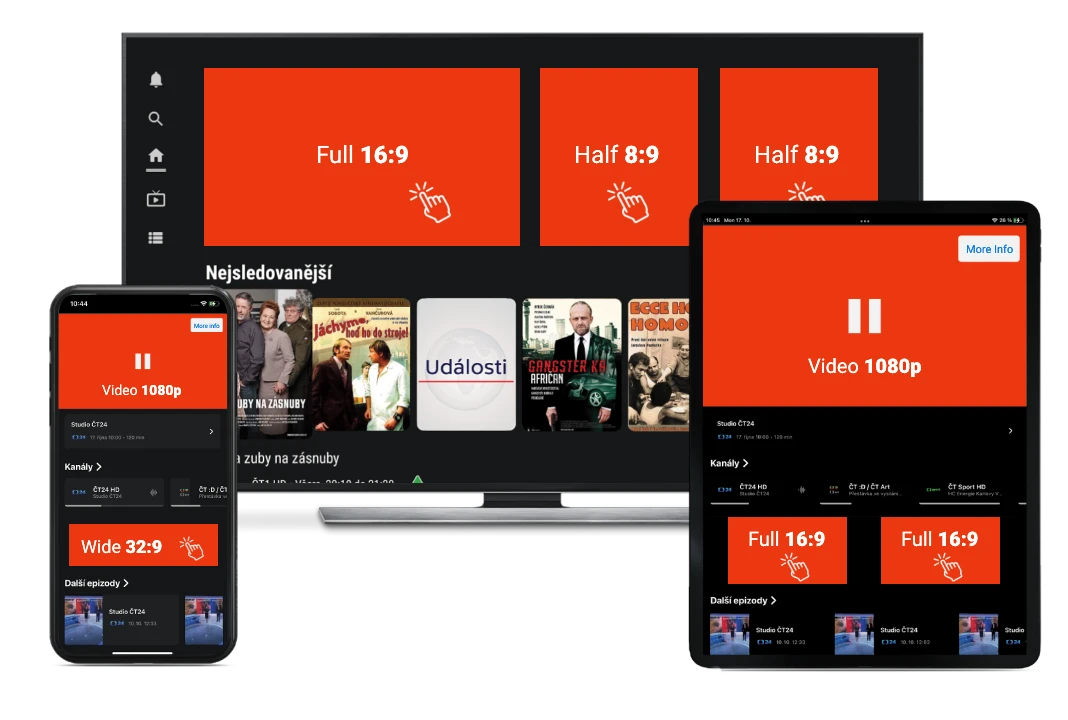## Unique Benefits

A/B testing possibilities

Targeting options

Great Price-Performance ratioBasic coefficient

1 = 900 CZK / CPT

Surcharge coefficients

TV channel targeting   +0.5

device targeting   +0.5

Option to skip Ad after 5 seconds.

If the Ad is skipped within 10 seconds, we subtract 0.3 from the total coefficient.

Please note that our prices are VAT exclusive.

( MPEG-4 (.mp4) 1920x1080 px )

 Duration Koeficient 5s -0.15 10s 1 every other 5s started +0.25

Static video Ad in the player

( jpg, png - 1920x1080 px )

 Duration Coefficient 3s -0.5

Examples of coefficient calculations

The price is calculated as follows: Ad duration (coefficient 1 - 0.5 = 0.5).

Total price is 0.5 x 900 = 450 CZK / CPT.

Video Ad duration is 5s without targeting.

The price is calculated as follows: Ad duration (coefficient 1 - 0.15 = 0.85).

Total price is 0.85 x 900 = 765 CZK / CPT.

Video Ad duration is 18s with targeting TV channel (e.g. CT Sport).

The price is calculated as follows: Ad duration (coefficient 1 + 0.25 + 0.25 = 1.5) + TV channel targeting (0.5).

Total price is 2 x 900 = 1 800 CZK / CPT.

Video Ad duration is 26s with targeting TV channel and device targeting.

The price is calculated as follows: Ad duration (coefficient 1 + 0.25 + 0.25 + 0.25 +0.25 = 2) + TV channel and device targeting (0.5 + 0.5 = 1).

Total price is 3 x 900 = 2 700 CZK / CPT.